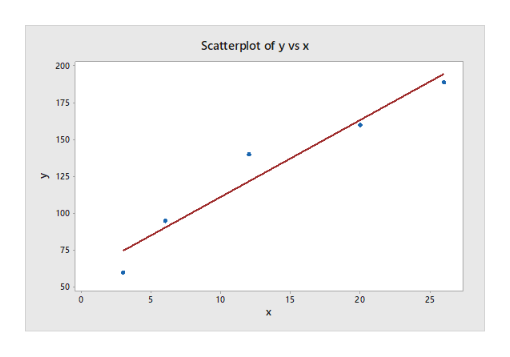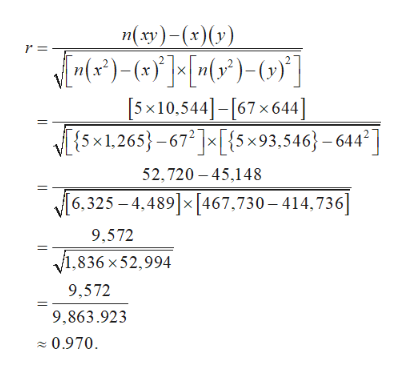# How much should a healthy Shetland pony weigh? Let x be the age of the pony (in months), and let y be the average weight of the pony (in kilograms).x36122026y6095140160189(a) Make a scatter diagram of the data and visualize the line you think best fits the data.  Flash Player version 10 or higher is required for this question. You can get Flash Player free from Adobe's website. (b) Would you say the correlation is low, moderate, or strong? lowmoderate    strongWould you say the correlation is positive or negative? positivenegative    (c) Use a calculator to verify that     x = 67,     x2 = 1265,     y = 644,     y2 = 93,546, and     xy = 10,544. Compute r. (Round your answer to three decimal places.)  As x increases from 3 to 26 months, does the value of r imply that y should tend to increase or decrease? Explain your answer. Given our value of r, y should tend to increase as x increases.Given our value of r, we can not draw any conclusions for the behavior of y as x increases.Given our value of r, y should tend to remain constant as x increases.Given our value of r, y should tend to decrease as x increases.

Question
848 views

How much should a healthy Shetland pony weigh? Let x be the age of the pony (in months), and let y be the average weight of the pony (in kilograms).

 x 3 6 12 20 26 y 60 95 140 160 189
(a) Make a scatter diagram of the data and visualize the line you think best fits the data.

Flash Player version 10 or higher is required for this question.
You can get Flash Player free from Adobe's website.

(b) Would you say the correlation is low, moderate, or strong?
low
moderate
strong

Would you say the correlation is positive or negative?
positive
negative

(c) Use a calculator to verify that
x = 67,

x2 = 1265,

y = 644,

y2 = 93,546,
and
xy = 10,544.

As x increases from 3 to 26 months, does the value of r imply that y should tend to increase or decrease? Explain your answer.
Given our value of ry should tend to increase as x increases.
Given our value of r, we can not draw any conclusions for the behavior of y as x increases.
Given our value of ry should tend to remain constant as x increases.
Given our value of ry should tend to decrease as x increases.
check_circle

Step 1

Part (a):

The scatter diagram, along with the best fitted line (least-squares regression line) for the data is as follows:help_outlineImage TranscriptioncloseScatterplot of y vs x 200 175 150 125 100 75 50 0 10 15 20 25 309 fullscreen
Step 2

Part (b):

All the points fall very close to the straight line in the graph, in such a way, that as x increases, y also increases.

Thus, the correlation is strong and positive.

Step 3

Part (c):

Here, x, x2, y, y2, and xy are the sums of X, X2 Y, Y2, and XY, respectively.

Using calculator, it is verified that:

x = (3 + 6 + 12 + 20 + 26) = 67.

x2 = (32 + 62 + 122 + 202 + 262) = 1,265.

y = (60 + 95 + 140 + 160 + 189) = 644.

y2 = (602 + 952 + 1402 + 1602 + 1892) = 93,546.

xy = (3×60) + (6×95) + (12×140) + (20×160) + (26×189) = 10...help_outlineImage Transcriptionclosenxy)-(x)(y) (*)(x] [n(*)-(y [5x10,544][67x 64] ESx1.265-672x[5x93.546}-644 52,720 45,148 6,325-4,489x[467,730-414,736] 9,572 V1,836 x52,994 9,572 9,863.923 0.970 fullscreen

### Want to see the full answer?

See Solution

#### Want to see this answer and more?

Solutions are written by subject experts who are available 24/7. Questions are typically answered within 1 hour.*

See Solution
*Response times may vary by subject and question.
Tagged in

### Data